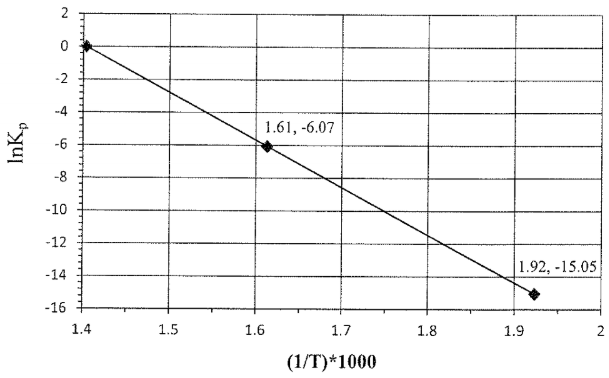# Problem: The temperature dependence of the equilibrium constant (Kp) is illustrated for the following reaction:NH4ClO4(s) → NH3(g) + HClO4(g)Note: the points are labeled as (1/T • 103, lnKp).Answer the following questions relating to the plot shown above:Calculate ΔH° (in kJ/mol) for the reaction given above. You may assume that ΔH° and ΔS° are temperature independent. Show all work and circle your final answer.

###### FREE Expert Solution

We’re being asked to determine the ΔH° using the provided data in the graph

This means we need to use the two-point form of the Arrhenius Equation:

where:

K1 = equilibrium constant at T1

K2 = equilibrium constant T

ΔH° = standard enthalpy

R = gas constant (8.314 J/mol•K)

T1 and T2 = temperature (in K).

85% (478 ratings)###### Problem Details

The temperature dependence of the equilibrium constant (Kp) is illustrated for the following reaction:

NH4ClO4(s) → NH3(g) + HClO4(g)

Note: the points are labeled as (1/T • 103, lnKp).Answer the following questions relating to the plot shown above:

Calculate ΔH° (in kJ/mol) for the reaction given above. You may assume that ΔH° and ΔS° are temperature independent. Show all work and circle your final answer.